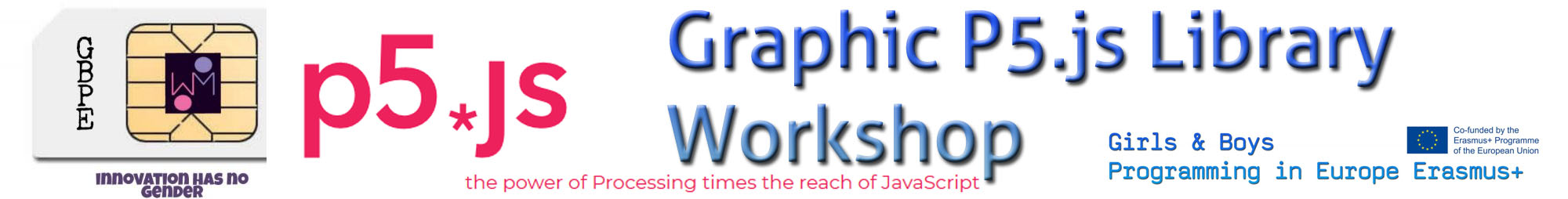## P5.js Workshop

This workshop about P5.js Javascript Framework for graphic design and games was developed in the first Mobility in Novi Jicin organized by Medelova High School and the collaboration of TIETO.

From this lines we want to thank them for their attentions.

360 Shot of this activity

Post from RICOH THETA. #theta360 – Spherical Image – RICOH THETA

We created a Brick Game with this code

``````// Global vars
var x = 300;
var y = 250;
var r = 10;
var dx = 3;
var dy = 1;
var c = 0;
var Psize=120;

function setup() {
createCanvas(400, 400);
}

function draw() {
background(0);
stroke(255,0,0);
strokeWeight(5);
ellipse(x, y, r + r, r + r);

//Update
x = x + dx;
y = y + dy;

// edges
if (x + r > width) {
dx = dx * -1;
}
if (y + r > height) {
dy = dy * -1;
}

if (x - r < 0) {
dx = dx * -1;
}
if (y - r < 0) {
dy = dy * -1;
}

// Drawing the Net
stroke(255);
for(let i=0;i<height;i+=5)  {
if(i%2==0)
{
line(width/2,i,width/2,i+5);
}
}

rectMode(CENTER);
var Py=constrain(mouseY,Psize/2,height-Psize/2);
stroke(0,255,0);
strokeWeight(5);
rect(20,Py,20,Psize);

if (x-r-r < 25   && (y-r > Py - Psize/2) && (y<Py+Psize/2) ) {
dx = dx * -1;
}

// ball reiniciate
if(x-r<0) {
reset();
}

}

function reset(){
x=width/2;
y=height/2
dx=2; dy=2;
}``````